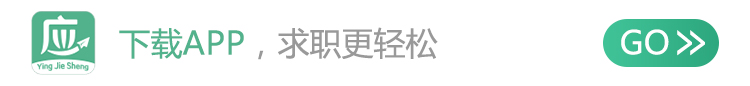# [湖北]招商银行武汉分行

r 就业指导中心电话：
r 卫津路校区：022-83613600；北洋园校区：022-27401081、27405214转8000
r r

r r

r r

r r

r r

r r

r r
1、定向培养生（FinTech方向
r r

r r

r r
2、定向培养生（业务类方向）
r r

r r

r r
3、联合培养生
r r

r r

r r
4、市场营销类岗位
r r

r r
5、运营支持类岗位
r r

r r
6、职能类岗位
r r

r r
7、业务类岗位（二级分行）
r r

r r

r r

r r
1、2020届毕业生（海外院校毕业时间需在2019年1月1日至2020年8月31日），全日制本科及以上学历（非定向），专业不限。
r r
2、诚实守信，性格开朗；崇尚专业，专注自律；积极乐观，抗压性强；拼搏创新，持续学习；无不良行为记录。
r r
3、身体健康，无影响胜任应聘岗位要求的疾病。
r r
4、具有较强的责任心、学习能力和良好的团队协作精神，具有良好的书面及口头表达能力。
r r
5、本科生英语四级考试分数425分（含）以上，研究生英语六级考试425分（含）以上。
r r
6、定向培养生（FinTech方向）须具备计算机、数学与统计、通信、物理等IT、DT相关专业背景。
r r

r r
1、招聘流程
r r

r r

r r
2、投递简历
r r

r r

r r

r r

r r
3、校园宣讲会
r r

r r
r r
r r 地点r r r 宣讲院校r r r 时间r r
r
r r 北京r r r 中国人民大学r r r 9月中旬r r
r
r r 北京r r r 北京航空航天大学r r r 9月中旬r r
r
r r 北京r r r 北京大学r r r 9月中旬r r
r
r r 武汉r r r 武汉大学r r r 9月中旬r r
r
r r 武汉r r r 华中科技大学r r r 9月中旬r r
r
r r 西安r r r 西安交通大学r r r 9月中旬r r
r
r r 厦门r r r 厦门大学r r r 9月中下旬r r
r
r r 天津r r r 南开大学r r r 9月中下旬r r
r
r r 四川r r r 四川大学r r r 9月中下旬r r
r
r r 广州r r r 中山大学r r r 9月下旬r r
r
r r 广州r r r 华南理工大学r r r 9月下旬r r
r
r r 北京r r r 北京理工大学r r r 10月上旬r r
r
r r 北京r r r 北京邮电大学r r r 10月上旬r r
r r
r r

r r

r r

r r
1、所有个人资料必须真实无误，如有虚假信息，申请人将会被取消申请资格；
r r
2、面试时间与招聘行程安排有关，敬请关注分行相关公告通知；
r r
3、当您的手机号码变更时，请及时更新注册信息，这是我们跟您联系的重要渠道；
r r
4、应聘渠道以招商银行官网网申为准。招商银行招聘不收取任何费用！
r r
5、联系我们：whcv@cmbchina.com（供咨询，简历请从招聘系统中投递喔~）。